Students can download Maths Chapter 2 Introduction to Algebra Ex 2.1 Questions and Answers, Notes, Samacheer Kalvi 6th Maths Guide Pdf helps you to revise the complete Tamilnadu State Board New Syllabus, helps students complete homework assignments and to score high marks in board exams.

## Tamilnadu Samacheer Kalvi 6th Maths Solutions Term 1 Chapter 2 Introduction to Algebra Ex 2.1

Question 1.
Fill in the blanks:
(i) The letters a, b, c, .., x, y, z are used to represent _____
(ii) A quantity that takes _____ values is called a variable.
(iii) If there are 5 students on a bench, then the number of students in ‘n’ benches is ‘5 × n’. Here _____ is a variable.
Solution:
(i) Variables
(ii) Different
(iii) nQuestion 2.
Say True or False.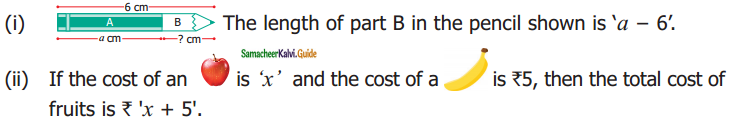(iii) If there are 11 players in a team, then there will be ’11 + q’ players in ‘q’ teams.
Solution:
(i) False
(ii) True
(iii) False

Question 3.
Draw the next two patterns and complete the table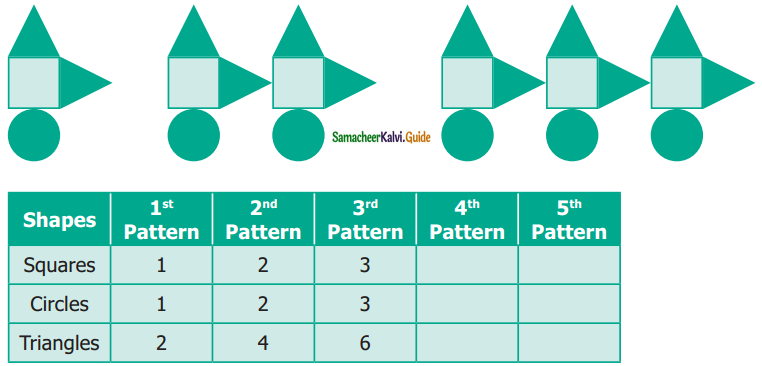Solution: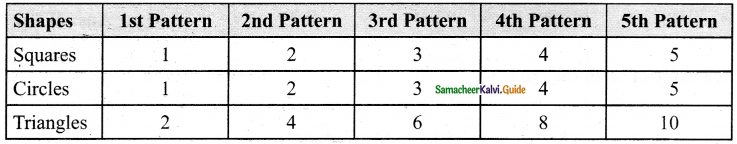Question 4.
Use a variable to write the rule, which gives the number of ice candy sticks required to make the following patterns.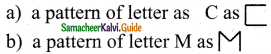Solution:
(a) No of ice candy sticks used = 3 × n = 3n
(b) No of ice candy sticks used = 4 × n = 4n

Question 5.
The teacher forms groups of five students in a class. How many students will be there in ‘p’ groups?
Solution:
No of students in a group = 5
No of students in ‘p’ groups = 5 × p = 5p

Question 6.
Arivazhagan is 30 years younger than his father. Write Arivazhagan’s age in terms of his father’s age.
Solution:
Let Arivazhagan’s father’s age be x years
According to the problem,
Arivazhagan’s age = (x – 30) years

Question 7.
If ‘u’ is an even number, how would you represent
(i) the next even number?
(ii) the previous even number?
Solution:
(i) Difference between two even numbers = 2
Given that ‘u’ is an even number.
Next, the even number is u + 2.
(ii) Previous even number is u – 2.

Objective Type Questions

Question 8.
Variable means that it
(a) can take only a few values
(b) has a fixed value
(c) can take different values
(d) can take only 8 values
Solution:
(c) can take different values

Question 9.
‘6y’ means
(a) 6 + y
(b) 6 – y
(c) 6 × y
(d) $$\frac{6}{7}$$
Solution:
(c) 6 × y

Question 10.
Radha is ‘x’ years of age now. 4 years ago, her age was
(a) x – 4
(b) 4 – x
(c) 4 + x
(d) 4x
Solution:
(a) x – 4

Question 11.
The number of days in ‘w’ weeks is
(a) 30 + w
(b) 30w
(c) 7 + w
(d) 7w
Solution:
(d) 7w

Question 12.
The value of ‘x’ in the circle is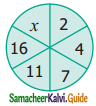(a) 6
(b) 8
(c) 21
(d) 22
Solution:
(d) 22